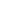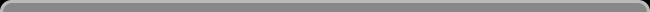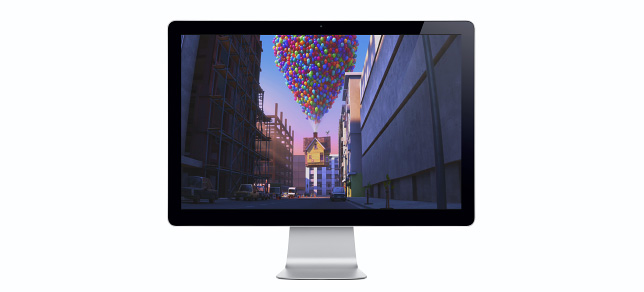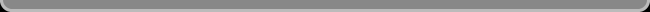Navigation: LaesieWorksImage Compression HomepageThis pageA 1 HOUR MOVIE

 A 1 hour movie in full high definition (1080p). That is a-lot-of data. How much exactly? When not compressed: - 24 bits for each RGB pixel (8 bits for each of the 3 primary light colors: Red, Green, and Blue) - A frame resolution of 1920 * 1080 = 2073600 pixels (16:9 widescreen) - A frame rate of 24 fps (24 images in each second) - One hour, that's 60*60 = 3600 seconds. - Not including: sound, subtitles, and such. The amount of bits is: 24 * 2073600 * 24 * 3600 = 4299816960000 bits The amount of 24 bits RGB pixels is: 2073600 * 24 * 3600 = 179159040000 RGB pixels The amount of 8 bits channel pixels is: 2073600 * 24 * 3 * 3600 = 537477120000 channel pixels The amount of frames is: 24 * 3600 = 86400 framesHow many GigaBytes is that? Calculated using 1024 per kilo: 4299816960000 / 8 / 1024 / 1024 / 1024 = 500.56 GB And that will NOT fit on one DVD (4.7 GB, or 8.5 GB dual layer) Nor will it fit on one Blu-ray disc! (25 GB, or 50 GB dual layer) To get this 1 hour movie on one dual layer Blu-ray disc, it must be compressed to even less than 50 GB, because the sound and such need to fit on the same disc. Let's say this movie may be no more than 20 GB large. From 500GB to only 20GB. That's a compression ratio of 500 / 20 = 25 The amount of bits that on average may be spend on 1 pixel is only: 24 / 25 = 0.96 bits How can that be done?! One RGB pixel needs 24 bits. One bit can make 1 out of 2 colors, while we need to get one out of 16777216 colors!

 MOVIE SPACE